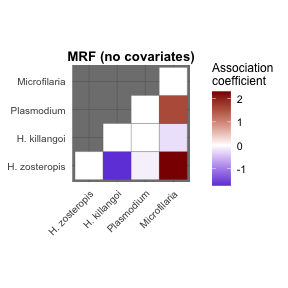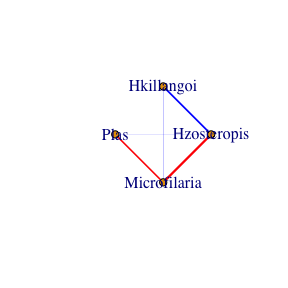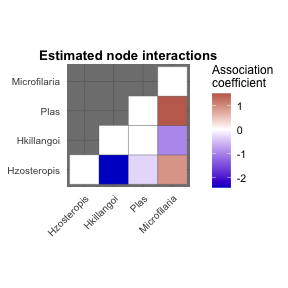# MRFs and CRFs for Bird.parasites data

We can explore the model’s primary functions using a test dataset that is available with the package. Load the Bird.parasites dataset, which contains binary occurrences of four avian blood parasites in New Caledonian Zosterops species (available in its original form at Dryad; Clark et al 2016). A single continuous covariate is also included (scale.prop.zos), which reflects the relative abundance of Zosterops species (compared to other avian species) among different sample sites

library(MRFcov)
data("Bird.parasites")

The Bird.parasites dataset is already in the appropriate structure for running MRFcov models; however, it is useful to understand how this was done. Using the raw dataset (downloaded from Dryad at the above link), we created a scaled continuous covariate to represent Zosterops spp. proportional captures in each sample site (as an estimate of relative abundance)

#Not run
#install.packages(dplyr)
data.paras = data.frame(data.paras) %>%
dplyr::group_by(Capturesession,Genus) %>%
dplyr::summarise(count = dlyr::n()) %>%
dplyr::mutate(prop.zos = count / sum(count)) %>%
dplyr::left_join(data.paras) %>%
dplyr::ungroup() %>% dplyr::filter(Genus == 'Zosterops') %>%
dplyr::mutate(scale.prop.zos = as.vector(scale(prop.zos)))
data.paras <- data.paras[, c(12:15, 23)]

You can visualise the dataset to see how analysis data needs to be structured. In short, for (family = "binomial") models, node variable (i.e. species) occurrences should be included as binary variables (1s and 0s) as the n_nodes left-most variables in data. Note that Gaussian continuous variables (family = "gaussian") and Poisson non-negative counts (family = "poisson") are also supported in MRFcov. Any covariates can be included as the right-most variables. It is recommended that these covariates all be on a similar scale, ideally using the scale function for continuous covariates (or similar) so that covariates have roughly mean = 0 and sd = 1, as this makes LASSO variable selection and comparisons of effect sizes very straightforward

help("Bird.parasites")
View(Bird.parasites)

### Running MRFs and visualising interaction coefficients

We first test for interactions using a Markov Random Fields (MRF) model without covariates. We set n_nodes as the number of species to be represented in the graphical model (4). We also need to specify the family for the model (binomial in this case). We do not specify the level of penalisation used by the LASSO algorithm. Instead, this is optimised separately for each species through cross validation (using function cv.glmnet in the glmnet package). This ensures the log-likelihood of each species is properly maximised before unifying them into an undirected graph. Finally, it is important to note that for MRF model functions, we can specify the number of processing cores to split the job across (i.e. n_cores). In this case we will only use 1 core, but higher numbers may increase speed (if more cores are available). Check available cores using the detectCores() function in the parallel package

MRF_fit <- MRFcov(data = Bird.parasites[, c(1:4)], n_nodes = 4, family = 'binomial')
## Leave-one-out cv used for the following low-occurrence (rare) nodes:
##  Microfilaria ...
## Warning: replacing previous import 'vctrs::data_frame' by 'tibble::data_frame'
## when loading 'dplyr'
## Warning: funs() was deprecated in dplyr 0.8.0.
## Please use a list of either functions or lambdas:
##
##   # Simple named list:
##   list(mean = mean, median = median)
##
##   # Auto named with tibble::lst():
##   tibble::lst(mean, median)
##
##   # Using lambdas
##   list(~ mean(., trim = .2), ~ median(., na.rm = TRUE))
## Fitting MRF models in sequence using 1 core ...

The message above can be useful for identifying nodes (i.e. species) that are very rare or very common, as these can be difficult to properly model. In this case, the Microfilaria node is fairly rare, and so the function automatically reverts to using leave-one-out cross-validation to optimise parameters. This can be slow, so be aware when attempting to use large datasets that contain many very rare or very common species. Now that the model has converged, we can plot the estimated interaction coefficients as a heatmap using plotMRF_hm. Note that we can specify the names of nodes should we want to change them

plotMRF_hm(MRF_mod = MRF_fit, main = 'MRF (no covariates)',
node_names = c('H. zosteropis', 'H. killangoi',
'Plasmodium', 'Microfilaria'))Note that other plotting methods an be used as desired. For instance, to plot these as a network instead, we can simply extract the adjacency matrix and plot it using standard igraph functions

net <- igraph::graph.adjacency(MRF_fit$graph, weighted = T, mode = "undirected") igraph::plot.igraph(net, layout = igraph::layout.circle, edge.width = abs(igraph::E(net)$weight),
edge.color = ifelse(igraph::E(net)$weight < 0, 'blue', 'red'))### Running CRFs using additional covariates We can now run a Conditional Random Fields (CRF) model using the provided continuous covariate (scale.prop.zos). Again, each species’ regression is optimised separately using LASSO regularization. Note that any columns in data to the right of column n_nodes will be presumed to represent covariates if we don’t specify an n_covariates argument MRF_mod <- MRFcov(data = Bird.parasites, n_nodes = 4, family = 'binomial') ## Leave-one-out cv used for the following low-occurrence (rare) nodes: ## Microfilaria ... ## Fitting MRF models in sequence using 1 core ... Visualise the estimated species interaction coefficients as a heatmap. These represent predicted interactions when the covariate is set to its mean (i.e. in this case, when scale.prop.zos = 0). If we had included other covariates, then this graph would represent interactions predicted when all covariates were set at their means plotMRF_hm(MRF_mod = MRF_mod)Regression coefficients and their relative importances can be accessed as well. This call returns a matrix of the raw coefficient, as well as standardised coefficients (standardised by the sd of the covariate). Standardisation in this way helps to compare the relative influences of each parameter on the target species’ occurrence probability, but in general the two coefficients will be identical (unless users have not pre-scaled their covariates). The list also contains contains each variable’s relative importance (calculated using the formula B^2 / sum(B^2), where the vector of Bs represents regression coefficients for predictor variables). Variables with an underscore (_) indicate an interaction between a covariate and another node, suggesting that conditional dependencies of the two nodes vary across environmental gradients. Because of this, it is recommended to avoid using column names with _ in them MRF_mod$key_coefs$Hzosteropis ## Variable Rel_importance Standardised_coef Raw_coef ## 1 Hkillangoi 0.67353426 -2.4332802 -2.4332802 ## 5 scale.prop.zos_Microfilaria 0.12348365 -1.0418783 -1.0418783 ## 3 Microfilaria 0.09499561 0.9138271 0.9138271 ## 4 scale.prop.zos 0.09327688 -0.9055226 -0.9055226 ## 2 Plas 0.01178155 -0.3218203 -0.3218203 Finally, a useful capability is to generate some fake data and test predictions. For instance, say we want to know how frequently malaria parasite infections are predicted to occur in sites with high occurrence of microfilaria fake.dat <- Bird.parasites fake.dat$Microfilaria <- rbinom(nrow(Bird.parasites), 1, 0.8)
fake.preds <- predict_MRF(data = fake.dat, MRF_mod = MRF_mod)

The returned object from predict_MRF depends on the family of the model. For family = "binomial", we get a list including both linear prediction probabilities and binary predictions (where a linear prediction probability > 0.5 equates to a binary prediction of 1). These binary predictions can be used to estimate parasite prevalence

H.zos.pred.prev <- sum(fake.preds$Binary_predictions[, 'Hzosteropis']) / nrow(fake.preds$Binary_predictions)
Plas.pred.prev <- sum(fake.preds$Binary_predictions[, 'Plas']) / nrow(fake.preds$Binary_predictions)
Plas.pred.prev
##  0.3474388

### Comparing fits of MRF and CRF models

A key step in the process of model exploration is to determine whether inclusion of covariates actually improves model fit and is warranted when estimating interaction parameters. This is straightforward for binomial models, as we can compare classification accuracy quickly and easily. The cv_diag functions will fit models and then determine their predictive performances against the supplied data. We can also compare MRF and CRF models directly to determine whether covariates are warranted. For this dataset, considering that parasite infections are quite rare, we are primarily interested in maximising model Sensitivity (capacity to successfully predict positive infections)

mod_fits <- cv_MRF_diag_rep(data = Bird.parasites, n_nodes = 4,
n_cores = 1, family = 'binomial', plot = F,
compare_null = T,
n_folds = 10)
## Generating node-optimised Conditional Random Fields model
##
## Generating Markov Random Fields model (no covariates)
##
## Calculating model predictions of the supplied data
## Generating CRF predictions ...
## Generating null MRF predictions ...
##
## Calculating predictive performance across test folds
## Processing cross-validation run 1 of 10 ...
## Processing cross-validation run 2 of 10 ...
## Processing cross-validation run 3 of 10 ...
## Processing cross-validation run 4 of 10 ...
## Processing cross-validation run 5 of 10 ...
## Processing cross-validation run 6 of 10 ...
## Processing cross-validation run 7 of 10 ...
## Processing cross-validation run 8 of 10 ...
## Processing cross-validation run 9 of 10 ...
## Processing cross-validation run 10 of 10 ...
# CRF (with covariates) model sensitivity
quantile(mod_fits$mean_sensitivity[mod_fits$model == 'CRF'], probs = c(0.05, 0.95))
##        5%       95%
## 0.1151709 0.4444444
# MRF (no covariates) model sensitivity
quantile(mod_fits$mean_sensitivity[mod_fits$model != 'CRF'], probs = c(0.05, 0.95))
##         5%        95%
## 0.03685185 0.27606897

### Bootstrapping the data and running models

We now may want to fit models to bootstrapped subsets of the data to account for parameter uncertainty. Users can change the proportion of observations to include in each bootstrap run with the sample_prop option.

booted_MRF <- bootstrap_MRF(data = Bird.parasites, n_nodes = 4, family = 'binomial', n_bootstraps = 10, n_cores = 1)
## Fitting bootstrap_MRF models in sequence using 1 core...

### Exploring regression coefficients and interpreting results

Finally, we can explore regression coefficients to get a better understanding of just how important interactions are for predicting species’ occurrence probabilities (in comparison to other covariates). This is perhaps the strongest property of CRFs, as comparing the relative importances of interactions and fixed covariates using competing methods (such as Joint Species Distribution Models) is difficult. The bootstrap_MRF function conveniently returns a matrix of important coefficients for each node in the graph, as well as their relative importances

booted_MRF$mean_key_coefs$Hzosteropis
##                      Variable Rel_importance  Mean_coef
## 1                  Hkillangoi     0.66977842 -2.3835559
## 5 scale.prop.zos_Microfilaria     0.12354997 -1.0237198
## 3                Microfilaria     0.09898651  0.9163213
## 4              scale.prop.zos     0.09275389 -0.8870045
## 2                        Plas     0.01203770 -0.3195446
booted_MRF$mean_key_coefs$Hkillangoi
##         Variable Rel_importance  Mean_coef
## 1    Hzosteropis     0.77948038 -2.3835559
## 2   Microfilaria     0.13109170 -0.9774855
## 3 scale.prop.zos     0.08942315 -0.8073237
booted_MRF$mean_key_coefs$Plas
##                      Variable Rel_importance  Mean_coef
## 2                Microfilaria     0.65173449  1.5114438
## 3              scale.prop.zos     0.26336942 -0.9608140
## 4 scale.prop.zos_Microfilaria     0.04875339  0.4133891
## 1                 Hzosteropis     0.02913062 -0.3195446
booted_MRF$mean_key_coefs$Microfilaria
##                     Variable Rel_importance  Mean_coef
## 3                       Plas     0.34854151  1.5114438
## 4             scale.prop.zos     0.19160871 -1.1206561
## 5 scale.prop.zos_Hzosteropis     0.15989419 -1.0237198
## 2                 Hkillangoi     0.14577773 -0.9774855
## 1                Hzosteropis     0.12810500  0.9163213
## 6        scale.prop.zos_Plas     0.02607286  0.4133891

Users can also use the predict_MRFnetworks function to calculate network statistics for each node in each observation or to generate adjacency matrices for each observation. By default, this function generates a list of igraph adjacency matrices, one for each row in data, which can be used to make network plots using a host of other packages. Note, both this function and the predict_MRF function rely on data that has a structure exactly matching to the data that was used to fit the model. In other words, the column names and column order need to be identical. The cutoff argument is important for binary problems, as this specifies the probability threshold for stating whether or not a species should be considered present at a site (and thus, whether their interactions will be present). Here, we state that a predicted occurrence above 0.33 is sufficient

adj_mats <- predict_MRFnetworks(data = Bird.parasites,
MRF_mod = booted_MRF,
metric = 'eigencentrality',
cutoff = 0.33)
apply(adj_mats, 2, summary)
##         Hzosteropis Hkillangoi      Plas Microfilaria
## Min.      0.0000000 0.00000000 0.0000000   0.00000000
## 1st Qu.   0.0000000 0.00000000 0.0000000   0.00000000
## Median    0.0000000 0.00000000 0.0000000   0.00000000
## Mean      0.1845723 0.07572383 0.1902671   0.08908686
## 3rd Qu.   0.4742848 0.00000000 0.1612472   0.00000000
## Max.      1.0000000 1.00000000 1.0000000   1.00000000

### Accounting for possible spatial autocorrelation

Lastly, MRFcov has the capability to account for possible spatial autocorrelation when estimating interaction parameters. To do this, we incorporate functions from the mgcv package to include smoothed Gaussian process spatial regression splines in each node-wise regression. The user must supply a two-column data.frame called coords, which will ideally contain Latitude and Longitude values for each row in data. We don’t have these coordinates for the Bird.parasites dataset, so we will instead create some fake coordinates to showcase the model. Note, these commands were not run here, but feel free to move through them as you did for the above examples

Latitude <- sample(seq(120, 140, length.out = 100), nrow(Bird.parasites), TRUE)
Longitude <- sample(seq(-19, -22, length.out = 100), nrow(Bird.parasites), TRUE)
coords <- data.frame(Latitude = Latitude, Longitude = Longitude)

The syntax for the MRFcov_spatial function is nearly identical to MRFcov, with the exception that coords must be supplied

CRFmod_spatial <- MRFcov_spatial(data = Bird.parasites, n_nodes = 4,
family = 'binomial', coords = coords)

Interpretation is also identical to MRFcov objects. Here, key coefficients are those that are retained after accounting for spatial influences

CRFmod_spatial$key_coefs$Hzosteropis

Finally, we can compare fits of spatial and non-spatial models just as we did for MRFs and CRFs above

cv_MRF_diag_rep_spatial(data = Bird.parasites, n_nodes = 4,
n_cores = 3, family = 'binomial', plot = T, compare_null = T,
coords = coords)